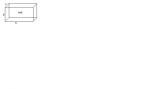# Pressure and resultant force

## Homework Statement

( athmosphere pressure is normal 101 kPa)
A ”tub” has a form as a prism ( like on the pic ) with the following lengths
Length: 2m
Width : 0,75m
height: 0,5m
Whats the absolute pressure agains the sides at the bottom of the “tub” + resultant forceI Think
p=po + pgh

## The Attempt at a Solution

(Im not sure about po and p )

For the absolute pressure I got :
P=Pa +pgh = 101 + 0,75*9,81*0,5 = 104,67 pa

And the resultant force
P = pgh = Resultantkraft: pgh = 0,75*9,81*0,5 = 3,675 pa
I have done someting wrong havent i ?

## Homework Statement

( athmosphere pressure is normal 101 kPa)
A ”tub” has a form as a prism ( like on the pic ) with the following lengths
Length: 2m
Width : 0,75m
height: 0,5m
Whats the absolute pressure agains the sides at the bottom of the “tub” + resultant forceI Think
p=po + pgh

## The Attempt at a Solution

(Im not sure about po and p )

For the absolute pressure I got :
P=Pa +pgh = 101 + 0,75*9,81*0,5 = 104,67 pa

And the resultant force
P = pgh = Resultantkraft: pgh = 0,75*9,81*0,5 = 3,675 pa
I have done someting wrong havent i ?

Someone Please im beggin heretiny-tim
Homework Helper
( athmosphere pressure is normal 101 kPa)
A ”tub” has a form as a prism ( like on the pic ) with the following lengths
Length: 2m
Width : 0,75m
height: 0,5m
Whats the absolute pressure agains the sides at the bottom of the “tub” + resultant force

For the absolute pressure I got :
P=Pa +pgh = 101 + 0,75*9,81*0,5 = 104,67 pa

And the resultant force
P = pgh = Resultantkraft: pgh = 0,75*9,81*0,5 = 3,675 pa

Hi Newton86!erm … what's the tub filled with?and I don't think the width affects the pressure, does it?Hi again TimIts filled with water. And yeah you got a point with the width :tongue:

I think I have it now after I see what pa and P is.

absolute pressure:
Pa +pgh = 101000 + 1000kg/m^3*9,81*0,5 = 105900 pa

101kpa is 101000 pa and 1000kg/m^3 is the density of water.

Resultant force:

Resultantkraft: pgh = 1000kg/m^3*9,81*0,5 = 4900 pa

does this make sense ?

tiny-tim
Homework Helper
Hi Newton86!Looks very good!(But I don't understand what they mean by "resultant force" … 49000pa is a pressure … pressure = force/area … do they mean resultant-force/area?)​

tiny-tim
Homework Helper
Hi Newton86!Looks very good!(But I don't understand what they mean by "resultant force" … 49000pa is a pressure … pressure = force/area … do they mean resultant-force/area?)​

Dont ask me You are the smart oneBut the resultant force to the bottom of the "tub"
I dont know but we got the information about lenght and width and so far i havent used that info, maybe i shouldtiny-tim
Hi Newton86!